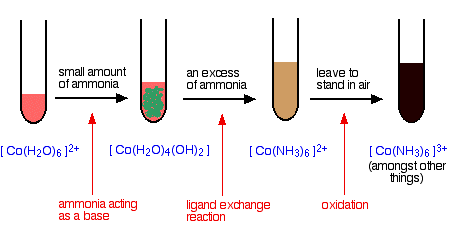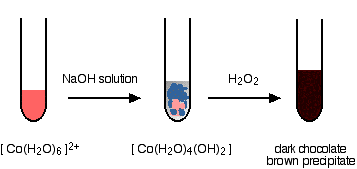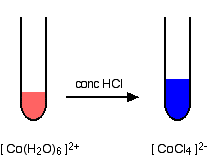Need help? Email help@shout.education

# Cobalt

This page looks at some aspects of cobalt chemistry required for UK A-level exams. These are mainly summarised from elsewhere on the site, with links available to more detailed explanations.

## Reactions of Cobalt(II) Ions in Solution

The simplest ion that cobalt forms in solution is the pink hexaaquacobalt(II) ion – [Co(H2O)6]2+.

Note: If you aren't happy about complex ions (including the way they are bonded and named), it would pay you to follow this link and explore the first couple of pages in the complex ions menu before you go on.

### The Reaction of Hexaaquacobalt(II) Ions With Hydroxide Ions

Hydroxide ions (from, say, sodium hydroxide solution) remove hydrogen ions from the water ligands attached to the cobalt ion.

Once a hydrogen ion has been removed from two of the water molecules, you are left with a complex with no charge – a neutral complex. This is insoluble in water and a precipitate is formed.

[\text{Co}(\text{H}_2\text{O})_6]^{2+} + \color{#467abf}{2\text{OH}^-} \longrightarrow [\text{Co}(\text{H}_2\text{O})_4(\text{OH})_2] + \color{#467abf}{2\text{H}_2\text{O}}

Note: The colour coding is to show that this isn't a ligand exchange reaction. The oxygens which were originally attached to the cobalt are still attached in the neutral complex.

In the test-tube, the colour changes are:Note: I don't know the reason for the change in colour. One reliable source quotes it vaguely as a change in coordination of the metal ion. Another suggests that the blue precipitate involves a chloride ion (from the cobalt(II) chloride solution if that's what you used) as a ligand. You are unlikely to need to worry about this at this level.

You will find the reactions between hexaaqua ions and hydroxide ions discussed in detail if you follow this link.

### Reactions of Hexaaquacobalt(II) Ions With Ammonia Solution

The ammonia acts as both a base and a ligand. With a small amount of ammonia, hydrogen ions are pulled off the hexaaqua ion exactly as in the hydroxide ion case to give the same neutral complex.

[\text{Co}(\text{H}_2\text{O})_6]^{2+} + 2\text{NH}_3 \longrightarrow [Co(\text{H}_2\text{O})_4(\text{OH})_2] + 2{\text{NH}_4}^+

That precipitate dissolves if you add an excess of ammonia. The ammonia replaces water as a ligand to give hexaamminecobalt(II) ions.

[\text{Co}(\text{H}_2\text{O})_6]^{2+} + 6\text{NH}_3 \longrightarrow [Co(\text{NH}_3)_6]^{2+} + 6\text{H}_2\text{O}

Note: You might wonder why this second equation is given starting from the original hexaaqua ion rather than the neutral complex. Explaining why the precipitate redissolves is quite complicated. You will find the explanation in full (although by reference to the corresponding copper case) on the page about the reactions between hexaaqua ions and ammonia solution.

The colour changes are:Note: With ammonia, the hydroxide precipitate is usually produced as a different colour from the one with sodium hydroxide solution (itself happening in two colour variations!). Obviously there is some re-complexing, but I don't know what it is.

The hexaamminecobalt(II) complex is very easily oxidised to the corresponding cobalt(III) complex. In the test-tube this is seen as a rapid darkening to a deep red-brown solution.

In fact the hexaamminecobalt(III) ion is yellow! What you see is a mixture of this ion and various other cobalt(III) ions involving ligand exchange reactions with both water molecules and negative ions present in the solution.

### Another Oxidation of Hexaaquacobalt(II) Ions

Rather than relying on oxidation by the air, you can add an oxidising agent such as hydrogen peroxide. You can do this after the addition of ammonia as in the last case, or you can do it following addition of sodium hydroxide solution.

#### With ammonia solution and hydrogen peroxide

The reaction with ammonia solution followed by hydrogen peroxide produces the same dark reddish-brown solution as before – only faster. The equation for the oxidation of the ammine complex is:

{2\text{Co}(\text{NH}_3)_6}^{2+} + \text{H}_2\text{O}_2 \longrightarrow {2\text{Co}(\text{NH}_3)_6}^{3+} + 2\text{OH}^-

Note: This equation is worked out in detail on one of the pages about writing ionic equations for redox reactions.

#### With sodium hydroxide solution and hydrogen peroxide

You get the variably coloured precipitate of the cobalt(II) hydroxide complex when you add the sodium hydroxide solution.

Addition of hydrogen peroxide produces lots of bubbles of oxygen and a dark chocolate brown precipitate.The final precipitate contains cobalt in the +3 oxidation state. I haven't given a formula for this, because I don't know exactly what it is! I can't give an equation for the same reason.

Note: One reliable source describes the precipitate as a "hydrous Co2O3" – in other words as cobalt(III) oxide with closely associated water. Another suggests a formula (in a similar, but not identical context) of CoO(OH). An internet search (to within the limits of my patience!) came up with nothing useful.

The oxygen seen in the reaction is produced from the decomposition of the hydrogen peroxide in a side reaction. Many things catalyse this decomposition – presumably, in this case, one or more of the various cobalt compounds present.

### The Reaction of Hexaaquacobalt(II) Ions With Carbonate Ions

You simply get a precipitate of what you can think of as cobalt(II) carbonate.

\text{Co}^{2+}_{(aq)} + {\text{CO}_3}^{2-}_{(aq)} \longrightarrow \text{CoCO}_{3(s)}Note: Only one of the UK A-level Exam Boards wants this, and this is the simplification that they make. In fact, the precipitate is better described as a basic carbonate with a formula of the type xCoCO3,yCo(OH)2,zH2O.

You will find the reactions between hexaaqua ions and carbonate ions discussed in detail if you follow this link.

### A Ligand Exchange Reaction Involving Chloride Ions

If you add concentrated hydrochloric acid to a solution containing hexaaquacobalt(II) ions, the solution turns from its original pink colour to a rich blue. The six water molecules are replaced by four chloride ions.The reaction taking place is reversible.

[\text{Co}(\text{H}_2\text{O})_6]^{2+} + 4\text{Cl}^- \xrightleftharpoons{} [\text{CoCl}_4]^{2-} + 6\text{H}_2\text{O}

If you add water to the blue solution, it returns to the pink colour.

Note: You will find this reaction explored in more detail on a page about ligand exchange reactions.